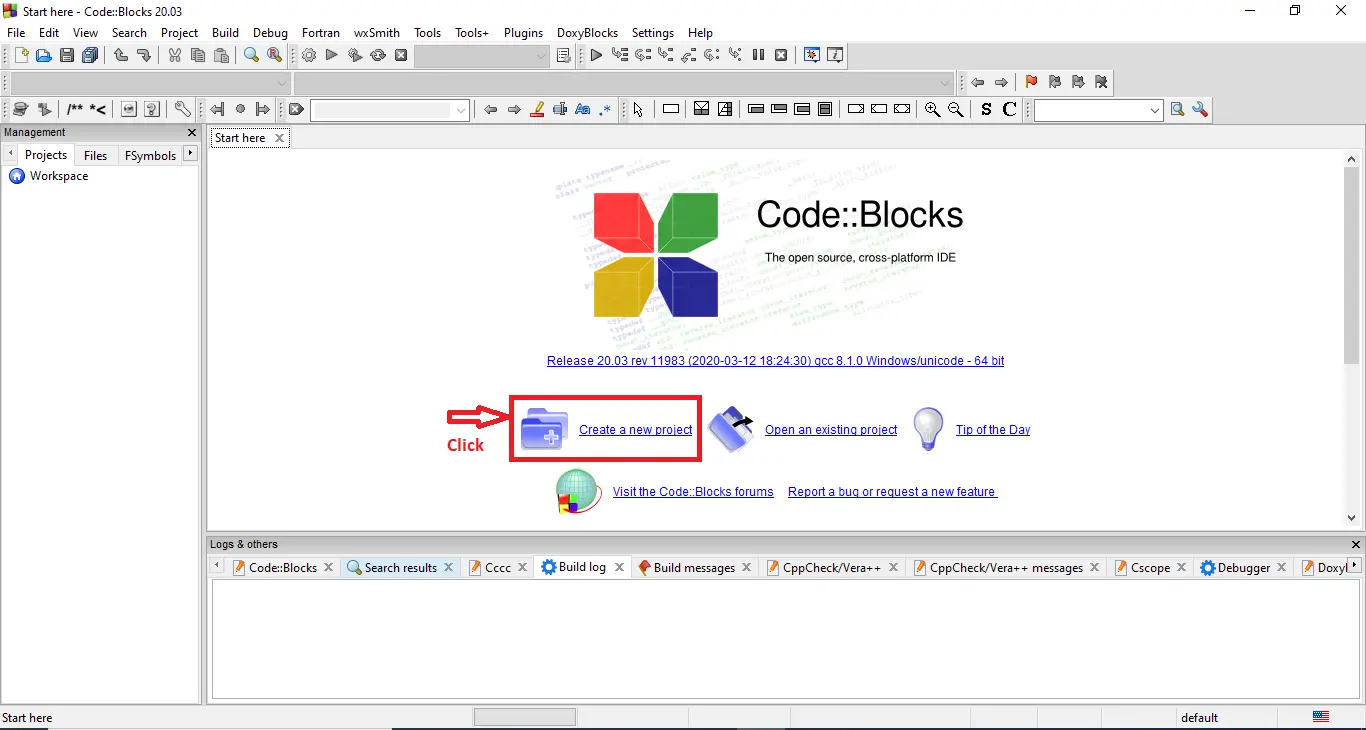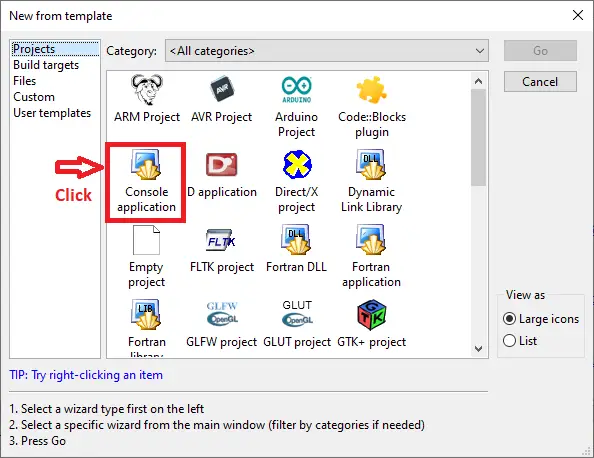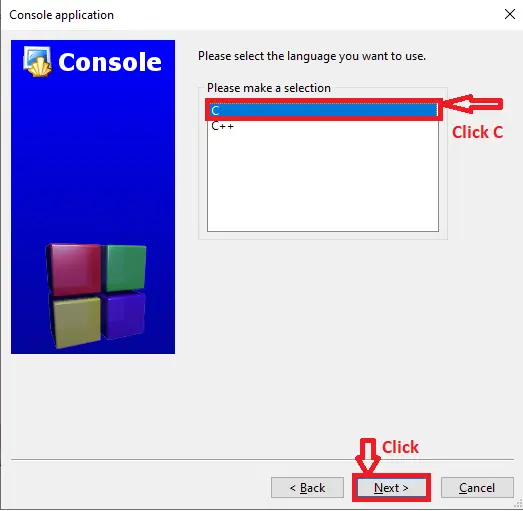# Multiplication Table in C with Source Code

## Multiplication Table in C with Source Code

This Multiplication Table Project in C teaches you how to create a simple Multiplication Table using computer programs. This is a modification to the previous software that generates the multiplication table up to a specific range (where range is also a positive integer entered by the user). You will learn how to generate the multiplication table of a number entered by the user in this example.

Multiplication Table Game Code in C Language it include a free Download Source Code. Simply locate the downloadable source code below and click the “Download Now” button. To start creating a Calculator in C Language, make sure that you have a Code Blocks or any platform of C installed in your computer.

## Multiplication Table in C Steps on How to Create a Project

Time needed: 5 minutes.

Here’s the step’s on how to create a Multiplication Table in C with Source Code.

• Step 1: Create a new project.

First open the code blocks IDE and click “create a new project“.• Step 2: Choose console application.

Next, click the “console application” and after that click “next“.• Step 3: Choose C language.

Then , choose “C language” and click “next“.• Step 4: Name your project.

Lastly, name the project you’ve created and click “next” after that click “finish“.• Step 5: The actual code.

Finally, we will now start adding functionality to our C Framework by adding some functional codes.

## This Multiplication Table in C was build and run under Code::Blocks IDE.

• Function to prompt the user for a positive range, we used a do while loop. The loop iterates again if the range value is negative, asking the user to provide a positive amount. We print the multiplication table once a positive range has been entered.(Multiplication Table Project in C)
```do {
printf("Enter the range (positive integer): ");
scanf("%d", &range);
} while (range <= 0);
```
• The user input is saved in the integer variable n in this case. Then we print the multiplication table up to ten using a for loop. From I = 1 through I = 10, the loop runs. n * I is printed in each iteration of the loop.(Multiplication Table Project in C)
```for (i = 1; i <= range; ++i) {
printf("%d * %d = %d \n", n, i, n * i);
}```